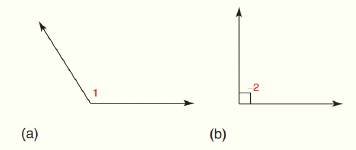Chapter 1.CR, Problem 18CR### Elementary Geometry for College St...

6th Edition
Daniel C. Alexander + 1 other
ISBN: 9781285195698

#### Solutions

Chapter
Section### Elementary Geometry for College St...

6th Edition
Daniel C. Alexander + 1 other
ISBN: 9781285195698
Textbook Problem
1 views

# On the basis of appearance, what type of angle is shown?To determine

a)

To find:

The type of angle on the basis of appearance.

Explanation

Given:

The given angle is shown as,

Figure (1)

Definition:

If the measure of an angle is less than 90° then that angle is known as acute angle.

If the measure of an angle is equal to 90° then that angle is known as right angle

To determine

b)

To find:

The type of angle on the basis of appearance.

### Still sussing out bartleby?

Check out a sample textbook solution.

See a sample solution

#### The Solution to Your Study Problems

Bartleby provides explanations to thousands of textbook problems written by our experts, many with advanced degrees!

Get Started

#### let f(x) = x3 + 5, g(x) = x2 2, and h(x)= 2x + 4. Find the rule for each function. 3. fg

Applied Calculus for the Managerial, Life, and Social Sciences: A Brief Approach

#### Differentiate the function. G(y)=ln(2y+1)5y2+1

Single Variable Calculus: Early Transcendentals

#### . 16.Minimize Subject to C=2x+3y x+y10x+2y122x+y12x0,y0

Finite Mathematics for the Managerial, Life, and Social Sciences

#### Evaluate 11(x2x3)dx a) 32 b) 56 c) 12 d) 23

Study Guide for Stewart's Single Variable Calculus: Early Transcendentals, 8th